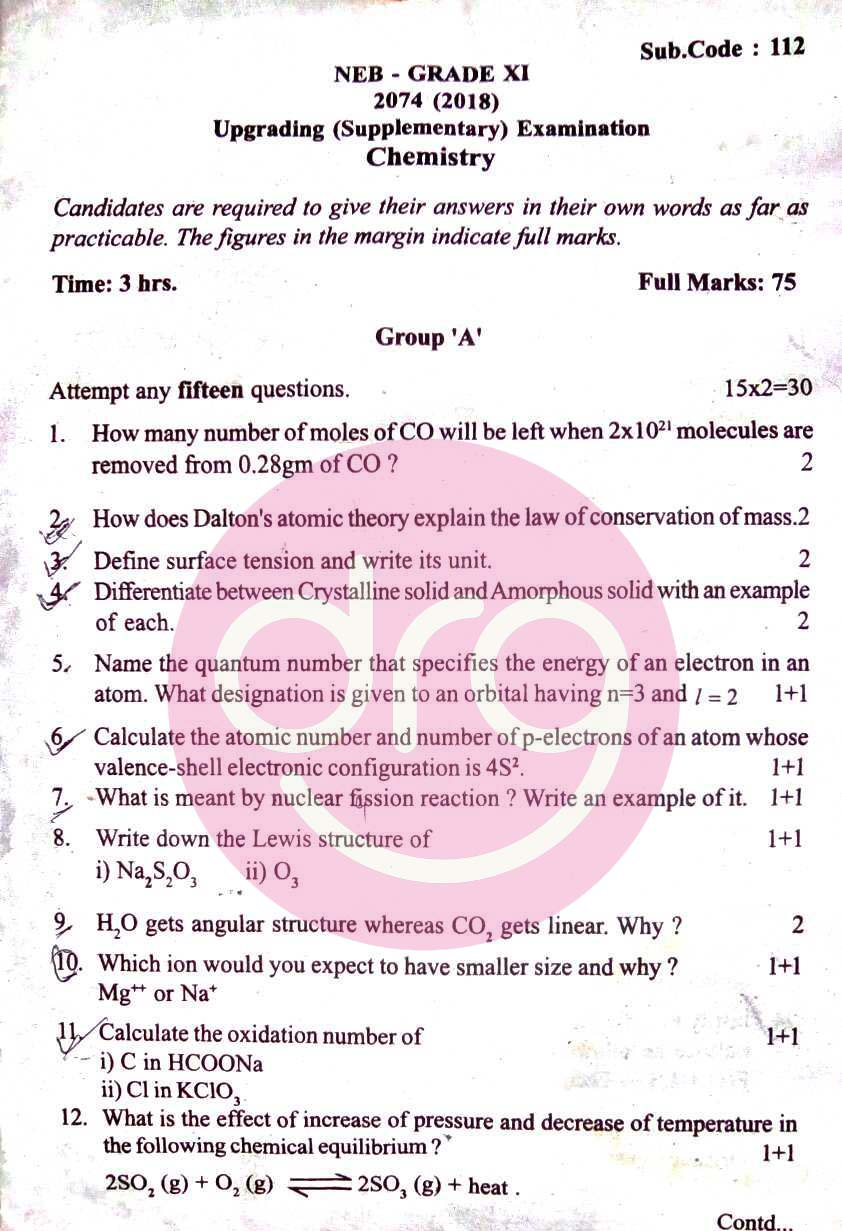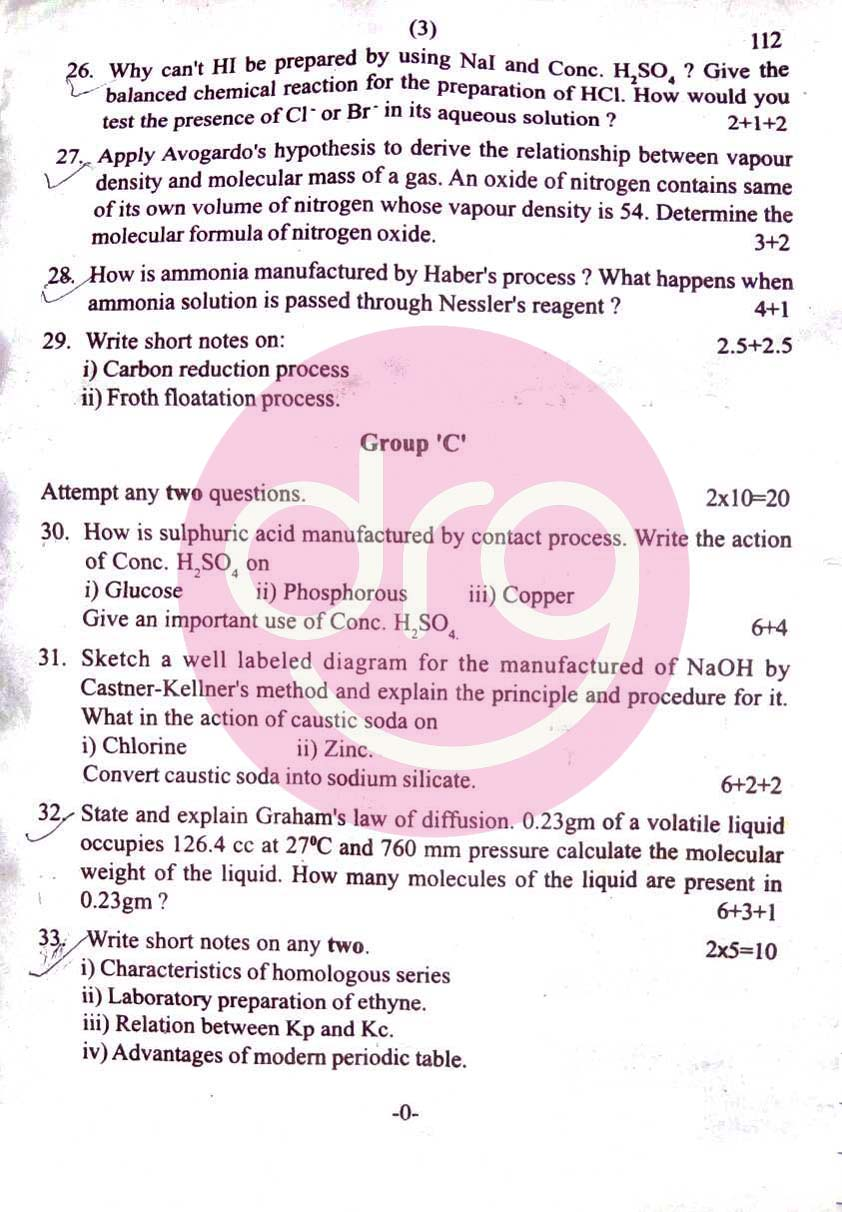NATIONAL EXAMINATIONS BOARD [NEB]
Examination - 2074 (2018)
Regular Students | Subject Code: 112
Time - 3 hrs
Full Marks - 75
Pass Marks - 27
Candidates are required to give their answers in their own words as far as practicable. The figures in the margin indicate full marks.
 Group 'A' Attempt any fifteen questions. [15x2=30] 1. How many numbers of moles of CO will be left when 2x1021 molecules are removed from 0.28gm of CO?  2. How does Dalton's atomic theory explain the law of conservation of mass.  3. Define surface tension and writes it unit. [1+1] 4. Differentiate between Crystalline solid and Amorphous with an example of each.  5. Name the quantum number that specifies the energy of an electron in an atom. What designation is given to an orbital having n=3 and ℓ=2.[1+1] 6. Calculate the atomic number and number of p-electrons of an atom whose valence shell electronic configuration is 4S2.  7. What is meant by nuclear fission reaction? Write an example of it. [1+1] 8. Write down the Lewis structure of  [1+1] i) N2S2O3     ii) O3 9. H2O gets angular structure whereas CO2 gets linear. Why?  10. Which ion would you expect to have smaller size and why? [1+1]        Mg++ or Na+ 11. Calculate the oxidation number of [1+1] i) C in HCOONa ii) Cl in KClO3  12. What is the effect of increase of pressure and decrease of temperature in the following chemical equilibrium? [1+1] i) 2SO2(g) + O2(g)⇌ 2SO3(g) + heat 13. Which one is more stable and why Ortho Hydrogen or Para Hydrogen. [1+1] 14. How are chlorofluorocarbon responsible for the depletion of ozone layer?  15. What is meant by allotropy? Name the hardest allotrope of carbon and it's use. [1+1] 16. Give a balanced chemical reaction for the preparation of PH3 in the laboratory when it is treated with O2. [1+1] 17. Define the terms: Gangue and Slag, giving proper example of each.  18. What happens when: [1+1] i) Gypsum salt is heated at 1200C ii) Chlorine gas is passed through slaked lime. 19. Write down IUPAC name of the following organic compounds. [1+1] 20. Write an example of each of the followings: [1+1] i) Bayer's test,     ii) Wurtz reaction 21. Identify the major products Ⓐ and Ⓑ in the following reaction sequence.
See More:-
View Chemistry Upgrading(Supplementary) Question Paper of 2074/2018 NEB:Upgrading/Supplementary Chemistry Question Paper 2074-2018 NEBUpgrading/Supplementary Chemistry Question Paper 2074-2018 NEBUpgrading/Supplementary Chemistry Question Paper 2074-2018 NEB